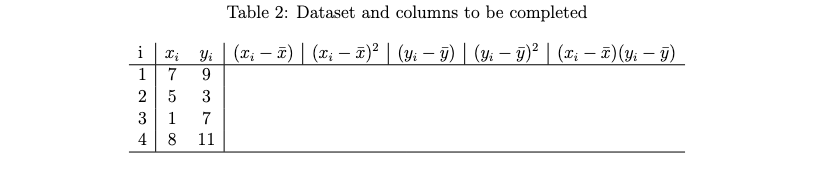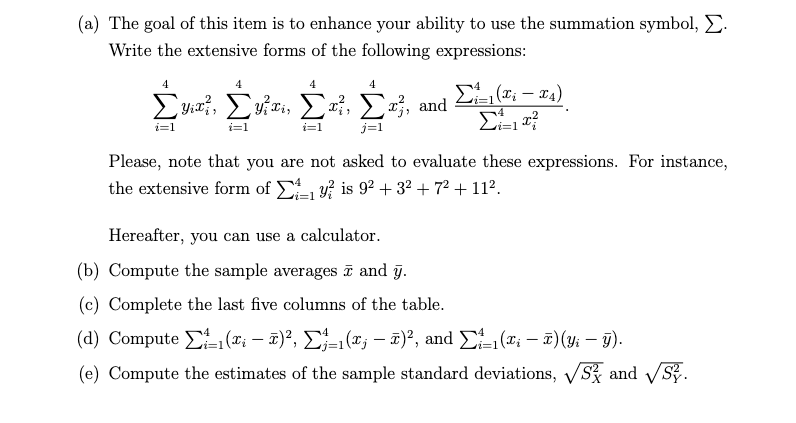Home / Expert Answers / Economics / table-2-dataset-and-columns-to-be-completed-a-the-goal-of-this-item-is-to-enhance-your-abili-pa779

# (Solved): Table 2 : Dataset and columns to be completed (a) The goal of this item is to enhance your abili ...Table 2 : Dataset and columns to be completed (a) The goal of this item is to enhance your ability to use the summation symbol, $$\sum$$. Write the extensive forms of the following expressions: $\sum_{i=1}^{4} y_{i} x_{i}^{2}, \sum_{i=1}^{4} y_{i}^{2} x_{i}, \sum_{i=1}^{4} x_{i}^{2}, \sum_{j=1}^{4} x_{j}^{2} \text {, and } \frac{\sum_{i=1}^{4}\left(x_{i}-x_{4}\right)}{\sum_{i=1}^{4} x_{i}^{2}} .$ Please, note that you are not asked to evaluate these expressions. For instance, the extensive form of $$\sum_{i=1}^{4} y_{i}^{2}$$ is $$9^{2}+3^{2}+7^{2}+11^{2}$$. Hereafter, you can use a calculator. (b) Compute the sample averages $$\bar{x}$$ and $$\bar{y}$$. (c) Complete the last five columns of the table. (d) Compute $$\sum_{i=1}^{4}\left(x_{i}-\bar{x}\right)^{2}, \sum_{j=1}^{4}\left(x_{j}-\bar{x}\right)^{2}$$, and $$\sum_{i=1}^{4}\left(x_{i}-\bar{x}\right)\left(y_{i}-\bar{y}\right)$$. (e) Compute the estimates of the sample standard deviations, $$\sqrt{S_{X}^{2}}$$ and $$\sqrt{S_{Y}^{2}}$$.

We have an Answer from Expert